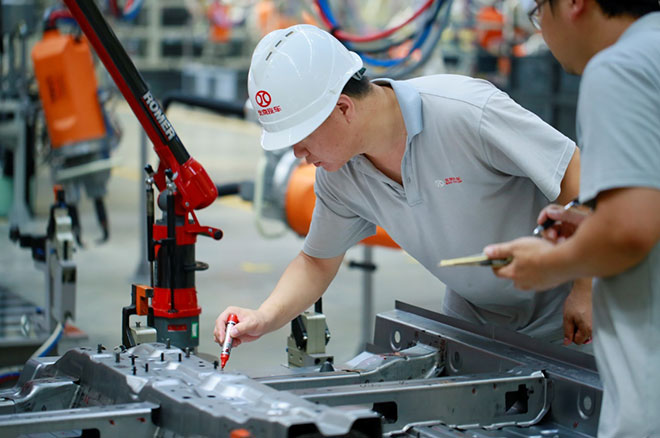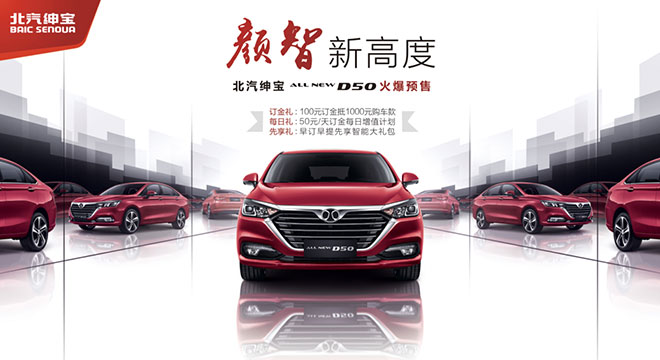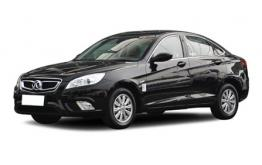# 北汽绅宝 绅宝D50

## 北京奔驰标准打造 全新绅宝D50量产下线全面预售

2017年09月26日 16:50 来源：车行天下 超过：14326次关注

9月26日，北汽绅宝2.0时代的开山之作——全新绅宝D50在誉为北汽绅宝智造基地的北京汽车株洲分公司正式量产下线。该基地参照北京奔驰标准打造，从制造设备到管理理念均达到业界领先水平，而这也保证了全新绅宝D50在品质方面具备叫板合资品牌的实力。在全新绅宝D50量产下线仪式现场，北京汽车股份有限公司总裁陈宏良表示：“全新绅宝D50的推出意味着北汽自主2.0时代全面开启，体现了北汽智造的高品质和前瞻性，新车全面满足新时代消费者对家轿的需求，必将引领自主品牌轿车的新高度。”

瞄准“中国制造2025”，北京汽车打造“诚意之作”

作为新生代的“颜智”派轿车，全新绅宝D50“高颜值”、“高智商”的背后，是高端工艺的加持和株洲分公司全体员工的匠心制造。全新绅宝D50的生产基地是参照“中国制造2025”战略智能制造标准、参照北京奔驰先进经验建设的全新工厂，即株洲分公司智造基地。其冲压设备具备整线一键自动切换模具功能，180秒切换完毕，设备检测精度可以达到0.03mm（相当于一根头发丝的直径）。焊接车间，采用了豪华车企标配的德国KUKA机器人，且机器人数达到176台，自动化率80%。涂装车间，使用领先行业的高泳透力电泳漆，可将车辆的防腐年限提升至10年。总装车间生产线的设备选型、检测设备及供应商，均对标德国汽车工业最高水准。而全新绅宝D50的生产制造队伍，是被北汽集团内部誉为“潇湘铁军”的株洲分公司团队。无论是早前的绅宝D20（E系列），还是此后的绅宝X25，多款单月销量过万的明星车型出自于此，相信全新绅宝D50必将成为下一个明星。采用全新设计语言，全新绅宝D50打造“颜智”标杆

全新绅宝D50自亮相起便备受关注，相继在上海车展、成都车展拿下了“最值得期待的新车”、“年度最具人气A级车”等多项大奖，可谓是“未上市就已先火”。

从造型设计层面来看，全新绅宝D50采用北汽绅宝最新的“OFFSPACE”设计语言，营造了无与伦比的“大都之美”:古典与时尚交融，恢弘与动感汇聚，凌厉与包容共生。细细打量，新车前脸显得大气时尚。侧面线条恢弘动感,再加上采纳光感曲面的设计理念、运动感十足的溜背设计,使整车造型彰显出“大都之美“的气质，遇见一部车，如遇一座城。作为一款新高度的“颜智”轿车，除了颜值，智能配置也是全新绅宝D50的一大亮点。新车搭载全新智能车机+语音控制系统，该系统不仅能识别普通话，更能识别十几种方言，语音拨号、语音导航、自定义车机唤醒等操作均可轻松实现。此外，全新绅宝D50配备主驾座椅6向电动调节、空气净化器等，充分考虑用户需求。

全新绅宝D50还致力追求安全“零死角”，除了坚固的笼式车身、全方位的安全气囊、ESP车身稳定系统，新车还搭载360度全景影像、盲区监测系统、车道偏离预警系统等高科技安全配置，产品实力远超同级别其他车型。动力方面，全新绅宝D50采用目前该级别主流的动力总成系统，其搭载高效节能的1.5L发动机，匹配手动和CVT两款变速箱。

值得一提的是，全新绅宝D50就曾经历过“高温、高寒、高原”三高极端环境的考验，先后“征服”了地表温度高达88℃的吐鲁番火焰山，海拔5000米的格尔木，零下40℃的呼伦贝尔等地，其卓越品质可见一斑。

作为北汽绅宝2.0时代的首款车型，全新绅宝D50的量产下线标志着生产制造达到阶段性目标，即将进入正式销售环节。据了解，全新绅宝D50在9月21日业已开启预售。在预售期间，前3000名订车并最终完成购车的客户，可享受丰厚的“三重大红包”：第一重“订金红包”，即100元订金抵1000元购车款。第二重“每日红包”，50元/天订金每日增值计划。即每距上市提前一天预订，便可节省50元车款。第三重“先享红包”，早订早提先享智能大礼包。顶级智造品质配合诚意满满的营销措施，使得全新绅宝D50成为超值之选，心动不如行动即日起可前往当地绅宝4S店进店咨询。

#### 相关文章

0-500 字已有评论 0条 查看评论>>

### 绅宝D50### 最新资讯

﻿
• 快速找车
• 选择品牌
• 选择品牌
• A  奥迪
• A  阿斯顿·马丁
• A  阿尔法·罗密欧
• B  宝沃
• B  布加迪
• B  巴博斯
• B  保时捷
• B  宾利
• B  奔驰
• B  宝马
• B  本田
• B  别克
• B  标致
• B  比亚迪
• B  宝骏
• B  北汽制造
• B  北汽新能源
• B  北汽幻速
• B  北汽威旺
• B  北京汽车
• B  奔腾
• B  北汽绅宝
• C  长安
• C  长安商用
• C  长城
• C  昌河
• D  大众
• D  道奇
• D  DS
• D  东南
• D  东风风神
• D  东风风行
• D  东风小康
• D  东风风度
• D  东风
• F  福特
• F  丰田
• F  菲亚特
• F  法拉利
• F  福田
• F  福迪
• F  福汽启腾
• G  观致
• G  广汽传祺
• G  广汽吉奥
• G  GMC
• H  红旗
• H  汉腾汽车
• H  哈弗
• H  哈飞
• H  海格
• H  海马
• H  华颂
• H  黄海
• H  华泰
• H  恒天
• J  吉利汽车
• J  捷豹
• J  Jeep
• J  江淮
• J  江铃
• J  金杯
• J  九龙
• J  金旅
• K  凯翼
• K  凯迪拉克
• K  克莱斯勒
• K  科尼塞克
• K  卡威
• K  开瑞
• L  路虎
• L  林肯
• L  劳斯莱斯
• L  兰博基尼
• L  雷克萨斯
• L  铃木
• L  雷诺
• L  理念
• L  力帆
• L  莲花汽车
• L  猎豹
• L  路特斯
• L  陆风
• M  马自达
• M  MG
• M  MINI
• M  玛莎拉蒂
• M  摩根
• M  迈凯轮
• N  纳智捷
• O  欧宝
• O  讴歌
• O  欧朗
• Q  奇瑞
• Q  起亚
• Q  启辰
• R  日产
• R  荣威
• R  瑞麒
• S  三菱
• S  斯威汽车
• S  萨博
• S  smart
• S  斯柯达
• S  斯巴鲁
• S  思铭
• S  双龙
• S  上汽大通
• S  双环
• T  特斯拉
• T  腾势
• W  沃尔沃
• W  五菱汽车
• W  五十铃
• W  威兹曼
• W  威麟
• X  现代
• X  雪佛兰
• X  雪铁龙
• X  西雅特
• Y  一汽
• Y  英菲尼迪
• Y  英致
• Y  依维柯
• Y  野马汽车
• Y  永源
• Z  众泰
• Z  中华
• Z  中兴
• Z  知豆
• 选择车系
• 选择车系
• 车型对比
• 选择品牌
• 选择品牌
• A  奥迪
• A  阿斯顿·马丁
• A  阿尔法·罗密欧
• B  宝沃
• B  布加迪
• B  巴博斯
• B  保时捷
• B  宾利
• B  奔驰
• B  宝马
• B  本田
• B  别克
• B  标致
• B  比亚迪
• B  宝骏
• B  北汽制造
• B  北汽新能源
• B  北汽幻速
• B  北汽威旺
• B  北京汽车
• B  奔腾
• B  北汽绅宝
• C  长安
• C  长安商用
• C  长城
• C  昌河
• D  大众
• D  道奇
• D  DS
• D  东南
• D  东风风神
• D  东风风行
• D  东风小康
• D  东风风度
• D  东风
• F  福特
• F  丰田
• F  菲亚特
• F  法拉利
• F  福田
• F  福迪
• F  福汽启腾
• G  观致
• G  广汽传祺
• G  广汽吉奥
• G  GMC
• H  红旗
• H  汉腾汽车
• H  哈弗
• H  哈飞
• H  海格
• H  海马
• H  华颂
• H  黄海
• H  华泰
• H  恒天
• J  吉利汽车
• J  捷豹
• J  Jeep
• J  江淮
• J  江铃
• J  金杯
• J  九龙
• J  金旅
• K  凯翼
• K  凯迪拉克
• K  克莱斯勒
• K  科尼塞克
• K  卡威
• K  开瑞
• L  路虎
• L  林肯
• L  劳斯莱斯
• L  兰博基尼
• L  雷克萨斯
• L  铃木
• L  雷诺
• L  理念
• L  力帆
• L  莲花汽车
• L  猎豹
• L  路特斯
• L  陆风
• M  马自达
• M  MG
• M  MINI
• M  玛莎拉蒂
• M  摩根
• M  迈凯轮
• N  纳智捷
• O  欧宝
• O  讴歌
• O  欧朗
• Q  奇瑞
• Q  起亚
• Q  启辰
• R  日产
• R  荣威
• R  瑞麒
• S  三菱
• S  斯威汽车
• S  萨博
• S  smart
• S  斯柯达
• S  斯巴鲁
• S  思铭
• S  双龙
• S  上汽大通
• S  双环
• T  特斯拉
• T  腾势
• W  沃尔沃
• W  五菱汽车
• W  五十铃
• W  威兹曼
• W  威麟
• X  现代
• X  雪佛兰
• X  雪铁龙
• X  西雅特
• Y  一汽
• Y  英菲尼迪
• Y  英致
• Y  依维柯
• Y  野马汽车
• Y  永源
• Z  众泰
• Z  中华
• Z  中兴
• Z  知豆
• 选择车系
• 选择车系
• 选择车型
• 选择车型
• 意见反馈